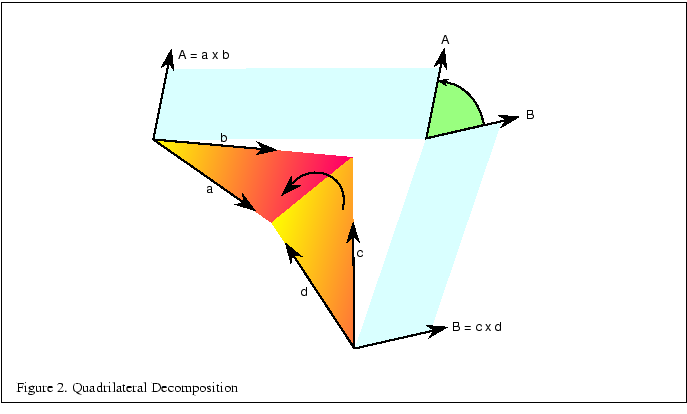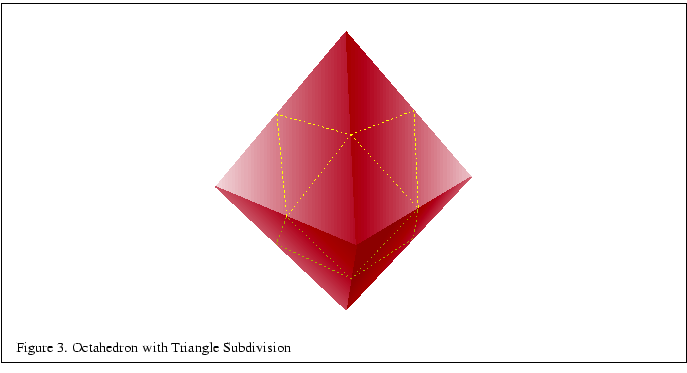Next: 3.3 Generating Model Normals Up: 3 Modeling Previous: 3.1 Modeling Considerations   Contents

## 3.2 Decomposition and Tessellation

Tessellation refers to the process of decomposing a complex surface such as a sphere into simpler primitives such as triangles or quadrilaterals. Most OpenGL implementations are tuned to process triangle strips and triangle fans efficiently. Triangles are desirable because they are planar, easy to rasterize, and can always be interpolated unambiguously. When an implementation is optimized for processing triangles, more complex primitives such as quad strips, quads, and polygons are decomposed into triangles early in the pipeline.

If the underlying implementation is performing this decomposition, there is a performance benefit in performing this decomposition a priori, either when the database is created or at application initialization time, rather than each time the primitive is issued. A second advantage of performing this decomposition under the control of the application is that the decomposition can be done consistently and independently of the OpenGL implementation. Since OpenGL does not specify its decomposition algorithm, different implementations may decompose a given quadrilateral along different diagonals. This can result in an image that is shaded differently and has different silhouette edges when drawn on two different OpenGL implementations.

Quadrilaterals may be decomposed by finding the diagonal that creates two triangles with the least difference in orientation. A good way to find this diagonal is to compute the angles between the normals at opposing vertices, compute the dot product, then choose the pair with the smallest angle (largest dot product) as shown in Figure 2. The normals for a vertex can be computed by taking the cross products of the the two vectors with origins at that vertex. An alternative decomposition method is to split the quadrilateral into triangles that are closest to equal in size.Tessellation of simple surfaces such as spheres and cylinders is not difficult. Most implementations of the GLU library use a simple latitude-longitude tessellation for a sphere. While the algorithm is simple to implement, it has the disadvantage that the triangles produced from the tessellation have widely varying sizes. These widely varying sizes can cause noticeable artifacts, particularly if the object is lit and rotating.

A better algorithm generates triangles with sizes that are more consistent. Octahedral and icosahedral tessellations work well and are not very difficult to implement. An octahedral tessellation approximates a sphere with an octahedron whose vertices are all on the unit sphere. Since the faces of the octahedron are triangles they can easily be split into four triangles, as shown in Figure 3.

Each triangle is split by creating a new vertex in the middle of each edge and adding three new edges. These vertices are scaled onto the unit sphere by dividing them by their distance from the origin (normalizing them). This process can be repeated as desired, recursively dividing all of the triangles generated in each iteration.The same algorithm can be applied using an icosahedron as the base object, recursively dividing all 20 sides. In both cases the algorithms can be coded so that triangle strips are generated instead of independent triangles, maximizing rendering performance. It is not necessary to split the triangle edges in half, since tessellating the triangle by other amounts, such as by thirds, or even any arbitrary number, may produce a more desirable final uniform triangle size.Next: 3.3 Generating Model Normals Up: 3 Modeling Previous: 3.1 Modeling Considerations   Contents
2001-01-10